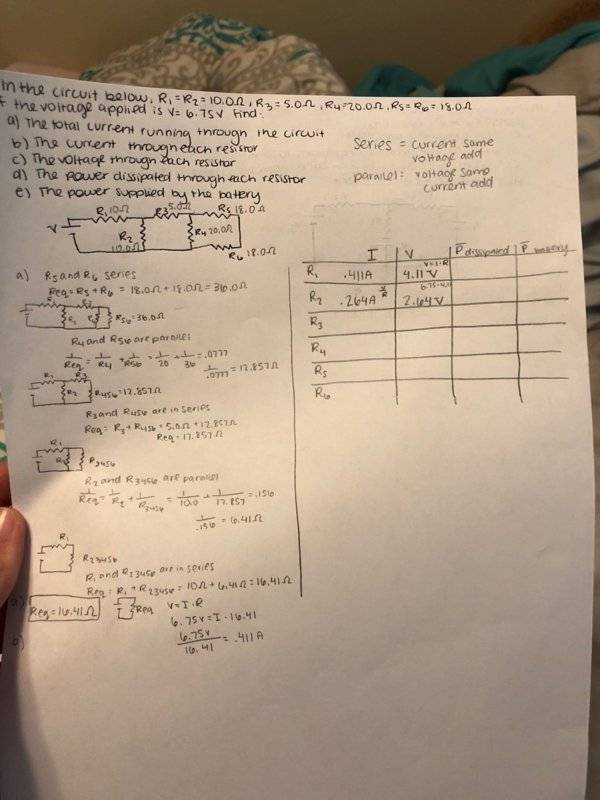# Finding the current and voltage for each resistor

prescripted

## Homework Statement

Moderators note: link removed. All images should be uploaded to PhysicsForums.## Homework Equations

V=IR
Series in parallel
Series in current

## The Attempt at a Solution

I managed to get the current and voltage of R1 and R2. I've been trying to get the next one R3, but haven't had success. I tried following the drawing, but couldn't figure out what I'm doing wrong. I thought that the current of R1 would be the current of R3. But I checked it against the solutions and it is incorrect.

#### Attachments

Last edited by a moderator:

## Answers and Replies

Homework Helper
The current through R2 plus R3 will equal the current in R1.
The current through R4 plus R5 will equal the current in R3.

Reading your work, all of your resistance calculations are correct.

•prescripted
Homework Helper
Your only mistake so far is thinking that the current through R1 and R3 are equal.

prescripted
Why does the current through R2 and R3 equal the current in R1? Does it have to do with the junction? And then I can't figure out R4 and R5 since I am unsure of what rule to follow. I figured out R3 would be I=.15, R=.75 .
Also, the reason I thought R1 and R3 current would be equal was because of the rule that in series currents are the same. But i guess R2 interrupts that rule?